# Simple and Exact Solutions to Position Calculation

#### 2019-03-06

This vignette contains solutions to various geographical position calculations. It is inspired and follows the 10 examples given at https://www.navlab.net/nvector/ .

Most of the content is based on (Gade 2010).

The color scheme in the Figures is as follows:

• $$\mathbf{\color{red}{Red}}$$: Given
• $$\mathbf{\color{green}{Green}}$$: Find this

## Example 1: A and B to delta

Given two positions $$A$$ and $$B$$, find the exact vector from $$A$$ to $$B$$ in meters north, east and down, and find the direction (azimuth/bearing) to $$B$$, relative to north. Use WGS-84 ellipsoid.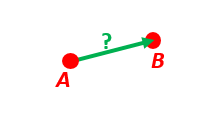A and B to delta.

### Solution

Transform the positions $$A$$ and $$B$$ to (decimal) degrees and depths:

# Position A:
z_EA <- 3

# Position B:
z_EB <- 6

Step 1: Convert to n-vectors, $$\mathbf{n}_{EA}^E$$ and $$\mathbf{n}_{EB}^E$$

(n_EA_E <- lat_lon2n_E(lat_EA, lon_EA))
#>  0.99923861 0.03489418 0.01745241
(n_EB_E <- lat_lon2n_E(lat_EB, lon_EB))
#>  0.99376802 0.08694344 0.06975647

Step 2: Find $$\mathbf{p}_{AB}^E$$ (delta decomposed in E). WGS-84 ellipsoid is default

(p_AB_E <-  n_EA_E_and_n_EB_E2p_AB_E(n_EA_E, n_EB_E, z_EA, z_EB))
#>  -34798.44 331985.66 331375.96

Step 3: Find $$\mathbf{R}_{EN}$$ for position $$A$$

(R_EN <- n_E2R_EN(n_EA_E))
#>               [,1]       [,2]        [,3]
#> [1,] -0.0174417749 -0.0348995 -0.99923861
#> [2,] -0.0006090802  0.9993908 -0.03489418
#> [3,]  0.9998476952  0.0000000 -0.01745241

Step 4: Find $$\mathbf{p}_{AB}^N = \mathbf{R}_{NE} \mathbf{p}_{AB}^E$$

# (Note the transpose of R_EN: The "closest-rule" says that when
# decomposing, the frame in the subscript of the rotation matrix that is
# closest to the vector, should equal the frame where the vector is
# decomposed. Thus the calculation R_NE*p_AB_E is correct, since the vector
# is decomposed in E, and E is closest to the vector. In the above example
# we only had R_EN, and thus we must transpose it: base::t(R_EN) = R_NE)
(p_AB_N <- base::t(R_EN) %*% p_AB_E %>%
as.vector())
#>  331730.23 332997.87  17404.27

Step 5: Also find the direction (azimuth) to $$B$$, relative to north

(azimuth <- atan2(p_AB_N, p_AB_N) %>%   # positive angle about down-axis
deg())
#>  45.10926

## Example 2: B and delta to C

A radar or sonar attached to a vehicle $$B$$ (Body coordinate frame) measures the distance and direction to an object $$C$$.

We assume that the distance and two angles (typically bearing and elevation relative to $$B$$) are already combined to the vector $$\mathbf{p}_{BC}^B$$ (i.e. the vector from $$B$$ to $$C$$, decomposed in B).

The position of $$B$$ is given as $$\mathbf{n}_{EB}^E$$ and $$z_{EB}$$, and the orientation (attitude) of $$B$$ is given as $$\mathbf{R}_{NB}$$ (this rotation matrix can be found from roll/pitch/yaw by using zyx2R).

Find the exact position of object $$C$$ as n-vector and depth ($$\mathbf{n}_{EC}^E$$ and $$z_{EC}$$), assuming Earth ellipsoid with semi-major axis $$a$$ and flattening $$f$$.

For WGS-72, use $$a = 6378135~\mathrm{m}$$ and $$f = \dfrac{1}{298.26}$$.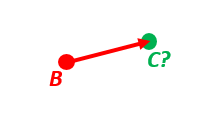B and delta to C.

### Solution

p_BC_B <- c(3000, 2000, 100)

# Position and orientation of B is given:
(n_EB_E <- unit(c(1, 2, 3))) # unit() to get unit length of vector
#>  0.2672612 0.5345225 0.8017837
z_EB <- -400
(R_NB <- zyx2R(rad(10),rad(20),rad(30))) # the three angles are yaw, pitch, and roll
#>            [,1]       [,2]       [,3]
#> [1,]  0.9254166 0.01802831  0.3785223
#> [2,]  0.1631759 0.88256412 -0.4409696
#> [3,] -0.3420201 0.46984631  0.8137977

# A custom reference ellipsoid is given (replacing WGS-84):
# (WGS-72)
a <- 6378135
f <- 1 / 298.26 

Step 1: Find $$\mathbf{R}_{EN}$$

(R_EN <- n_E2R_EN(n_EB_E))
#>            [,1]       [,2]       [,3]
#> [1,] -0.3585686 -0.8944272 -0.2672612
#> [2,] -0.7171372  0.4472136 -0.5345225
#> [3,]  0.5976143  0.0000000 -0.8017837

Step 2: Find $$\mathbf{R}_{EB}$$ from $$\mathbf{R}_{EN}$$ and $$\mathbf{R}_{NB}$$

(R_EB <- R_EN %*% R_NB) # Note: closest frames cancel
#>            [,1]       [,2]        [,3]
#> [1,] -0.3863656 -0.9214254  0.04119242
#> [2,] -0.4078587  0.1306225 -0.90365318
#> [3,]  0.8272684 -0.3659411 -0.42627939

Step 3: Decompose the delta vector $$\mathbf{p}_{BC}^B$$ in E

(p_BC_E <- R_EB %*% p_BC_B) # no transpose of R_EB, since the vector is in B)
#>           [,1]
#> [1,] -2997.828
#> [2,] -1052.696
#> [3,]  1707.295

Step 4: Find the position of $$C$$, using the functions that goes from one position and a delta, to a new position

l <- n_EA_E_and_p_AB_E2n_EB_E(n_EB_E, p_BC_E, z_EB, a, f)
(n_EB_E <- l[['n_EB_E']])
#>  0.2667916 0.5343565 0.8020507
(z_EB <- l[['z_EB']])
#>  -406.0072

Convert to latitude and longitude, and height

lat_lon_EB <- n_E2lat_lon(n_EB_E)
(latitude  <- lat_lon_EB)
#>  0.9307209
(longitude <- lat_lon_EB)
#>  1.107728

# height (= - depth)
(height <- -z_EB)
#>  406.0072

## Example 3: ECEF-vector to geodetic latitude

Position $$B$$ is given as an “ECEF-vector” $$\mathbf{p}_{EB}^E$$ (i.e. a vector from E, the center of the Earth, to $$B$$, decomposed in E).

Find the geodetic latitude, longitude and height (latEB, lonEB and hEB), assuming WGS-84 ellipsoid.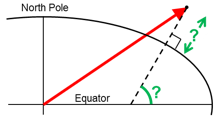ECEF-vector to geodetic latitude.

Position $$B$$ is given as $$\mathbf{p}_{EB}^E$$, i.e. “ECEF-vector”

(p_EB_E <- 6371e3 * c(0.9, -1, 1.1)) # m
#>   5733900 -6371000  7008100

### Solution

Find n-vector from the p-vector

l <- p_EB_E2n_EB_E(p_EB_E)
(n_EB_E <- l[['n_EB_E']])
#>   0.5170890 -0.5745433  0.6344439
(z_EB <- l[['z_EB']])
#>  -4702060

Convert to latitude and longitude, and height

lat_lon_EB <- n_E2lat_lon(n_EB_E)
(latEB  <- lat_lon_EB)
#>  0.6872888
(lonEB <- lat_lon_EB)
#>  -0.8379812

# height (= - depth)
(hEB <- -z_EB)
#>  4702060

## Example 4: Geodetic latitude to ECEF-vector

Find the ECEF-vector $$\mathbf{p}_{EB}^E$$ for the geodetic position $$B$$ given as latitude $$lat_{EB}$$, longitude $$lon_{EB}$$ and height $$h_{EB}$$.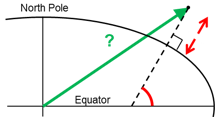Geodetic latitude to ECEF-vector.

### Solution

lat_EB <- rad(1)
h_EB <- 3

Step 1: Convert to n-vector

(n_EB_E <- lat_lon2n_E(lat_EB, lon_EB))
#>  0.99923861 0.03489418 0.01745241

Step 2: Find the ECEF-vector p_EB_E

(p_EB_E <- n_EB_E2p_EB_E(n_EB_E, -h_EB))
#>  6373290.3  222560.2  110568.8

## Example 5: Surface distance

Given two positions $$A$$ $$\mathbf{n}_{EA}^E$$ and $$B$$ $$\mathbf{n}_{EB}^E$$, find the surface distance $$s_{AB}$$ (i.e. great circle distance). The heights of $$A$$ and $$B$$ are not relevant (i.e. if they don’t have zero height, we seek the distance between the points that are at the surface of the Earth, directly above/below $$A$$ and $$B$$). Also find the Euclidean distance (chord length) $$d_{AB}$$ using nonzero heights.

Assume a spherical model of the Earth with radius $$r_{Earth} = 6371~\mathrm{km}$$.

Compare the results with exact calculations for the WGS-84 ellipsoid.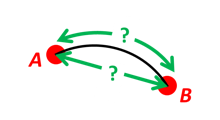Surface distance.

### Solution

n_EA_E <- lat_lon2n_E(rad(88), rad(0));
r_Earth <- 6371e3

#### Spherical model

The great circle distance is given by equations (16) in (Gade 2010) (the $$\arccos$$ is ill conditioned for small angles; the $$\arcsin$$ is ill-conditioned for angles near $$\pi/2$$, and not valid for angles greater than $$\pi/2$$) where $$r_{roc}$$ is the radius of curvature, i.e. Earth radius + height:

\begin{align} s_{AB} & = r_{roc} \cdot \arccos \!\big(\mathbf{n}_{EA}^E \boldsymbol{\cdot} \mathbf{n}_{EB}^E\big)\\ & = r_{roc} \cdot \arcsin \!\big(\big|\mathbf{n}_{EA}^E \boldsymbol{\times} \mathbf{n}_{EB}^E\big|\big) \tag{16} \end{align}

The formulation via $$\operatorname{atan2}$$ of equation (6) in (Gade 2010) is instead well conditioned for all angles:

$$s_{AB} = r_{roc} \cdot \operatorname{atan2}\big(\big|\mathbf{n}_{EA}^E \boldsymbol{\times} \mathbf{n}_{EB}^E\big|, \mathbf{n}_{EA}^E \boldsymbol{\cdot} \mathbf{n}_{EB}^E\big) \tag{6}$$

(s_AB <- (atan2(base::norm(pracma::cross(n_EA_E, n_EB_E), type = "2"),
pracma::dot(n_EA_E, n_EB_E)) * r_Earth))
#>  332456.4

The Euclidean distance is given by

$$d = r_{roc} \cdot \big| \mathbf{n}_{EB}^E - \mathbf{n}_{EA}^E \big|$$

(d_AB <- base::norm(n_EB_E - n_EA_E, type = "2") * r_Earth)
#>  332418.7

#### Elliptical model (WGS-84 ellipsoid)

The distance between $$A$$ and $$B$$ ca be calculated via geosphere package

geosphere::distGeo(c(0, 88), c(-170, 89))
#>  333947.5

## Example 6: Interpolated position

Given the position of $$B$$ at time $$t_0$$ and $$t_1$$, $$\mathbf{n}_{EB}^E(t_0)$$ and $$\mathbf{n}_{EB}^E(t_1)$$.

Find an interpolated position at time $$t_i$$, $$\mathbf{n}_{EB}^E(t_i)$$. All positions are given as n-vectors.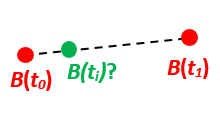Interpolated position.

### Solution

Standard interpolation can be used directly with n-vector as

$\mathbf{n}_{EB}^E(t_i) = \operatorname{unit}\Bigg(\mathbf{n}_{EB}^E(t_0) + \frac{t_i − t_0}{t_1 − t_0} \Big(\mathbf{n}_{EB}^E(t_1) − \mathbf{n}_{EB}^E(t_0)\Big)\Bigg)$

n_EB_E_t0 <- lat_lon2n_E(rad(89.9), rad(-150))

# The times are given as:
t0 <- 10
t1 <- 20
ti <- 16 # time of interpolation

Using the expression above

t_frac <- (ti - t0) / (t1 - t0)
(n_EB_E_ti <- unit(n_EB_E_t0 + t_frac * (n_EB_E_t1 - n_EB_E_t0) ))
#>  -0.0015114993  0.0001745329  0.9999988425

and converting back to longitude and latitude

(l  <- n_E2lat_lon(n_EB_E_ti) %>% deg())
#>   89.91282 173.41322
(latitude  <- l)
#>  89.91282
(longitude <- l)
#>  173.4132

## Example 7: Mean position (center/midpoint)

Given three positions $$A$$, $$B$$, and $$C$$ as n-vectors $$\mathbf{n}_{EA}^E$$, $$\mathbf{n}_{EB}^E$$, and $$\mathbf{n}_{EC}^E$$, find the mean position, $$M$$, as n-vector $$\mathbf{n}_{EM}^E$$.

Note that the calculation is independent of the depths of the positions.Mean position (center/midpoint).

### Solution

The (geographical) mean position $$B_{GM}$$ is simply given equation (17) in (Gade 2010) (assuming spherical Earth)

$\mathbf{n}_{EB_{GM}}^E = \operatorname{unit}\Big( \sum_{i = 1}^{m} \mathbf{n}_{EB_i}^E \Big) \tag{17}$

and specifically for the three given points

$\mathbf{n}_{EM}^E = \mathrm{unit}\Big(\mathbf{n}_{EA}^E + \mathbf{n}_{EB}^E + \mathbf{n}_{EC}^E \Big) = \frac{\mathbf{n}_{EA}^E + \mathbf{n}_{EB}^E + \mathbf{n}_{EC}^E}{\Big | \mathbf{n}_{EA}^E + \mathbf{n}_{EB}^E + \mathbf{n}_{EC}^E \Big| }$ Given the three n-vectors

n_EA_E <- lat_lon2n_E(rad(90), rad(0))
n_EC_E <- lat_lon2n_E(rad(50), rad(-20))

find the horizontal mean position

(n_EM_E <- unit(n_EA_E + n_EB_E + n_EC_E))
#>   0.38411717 -0.04660241  0.92210749

and convert to longitude/latitude

(l  <- n_E2lat_lon(n_EM_E) %>% deg())
#>  67.236153 -6.917511
(latitude  <- l)
#>  67.23615
(longitude <- l)
#>  -6.917511

## Example 8: A and azimuth/distance to B

Given a position $$A$$ as n-vector $$\mathbf{n}_{EA}^E$$, an initial direction of travel as an azimuth (bearing), $$\alpha$$, relative to north (clockwise), and finally the distance to travel along a great circle, $$s_{AB}$$ find the destination point $$B$$, given as $$\mathbf{n}_{EB}^E$$.

Use Earth radius $$r_{Earth}$$.

In geodesy this is known as “The first geodetic problem” or “The direct geodetic problem” for a sphere, and we see that this is similar to Example 2, but now the delta is given as an azimuth and a great circle distance. (“The second/inverse geodetic problem” for a sphere is already solved in Examples 1 and 5.)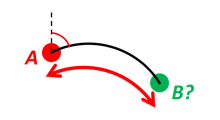A and azimuth/distance to B.

### Solution

Given the initial values

n_EA_E <- lat_lon2n_E(rad(80),rad(-90))
s_AB <- 1000 # distance (m)
r_Earth <- 6371e3 # mean Earth radius (m)

Step 1: Find unit vectors for north and east as per equations (9) and (10) in (Gade 2010)

\begin{align} \mathbf{k}_{east}^E & = \begin{bmatrix} 1 \\ 0 \\ 0 \end{bmatrix} \times \mathbf{n}^E \tag{9} \\ \mathbf{k}_{north}^E & = \mathbf{n}^E \times \begin{bmatrix} 1 \\ 0 \\ 0 \end{bmatrix} \times \mathbf{n}^E \tag{10} \end{align}

k_east_E <- unit(pracma::cross(base::t(R_Ee()) %*% c(1, 0, 0) %>% as.vector(), n_EA_E))
k_north_E <- pracma::cross(n_EA_E, k_east_E)

Step 2: Find the initial direction vector $$d_E$$

d_E <- k_north_E * cos(azimuth) + k_east_E * sin(azimuth)

Step 3: Find $$\mathbf{n}_{EB}^E$$

n_EB_E <- n_EA_E * cos(s_AB / r_Earth) + d_E * sin(s_AB / r_Earth)

Convert to longitude/latitude

(l  <- n_E2lat_lon(n_EB_E) %>% deg())
#>   79.99155 -90.01770
(latitude  <- l)
#>  79.99155
(longitude <- l)
#>  -90.0177

## Example 9: Intersection of two paths

Define a path from two given positions (at the surface of a spherical Earth), as the great circle that goes through the two points.

Path A is given by $$A_1$$ and $$A_2$$, while path B is given by $$B_1$$ and $$B_2$$.

Find the position C where the two great circles intersect.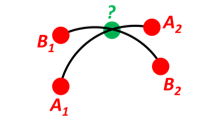Intersection of two paths.

### Solution

n_EA1_E <- lat_lon2n_E(rad(50), rad(180))

# These are from the python version (results are the same ;-)
# n_EB2_E <- lat_lon2n_E(rad(70), rad(80))

Find the intersection between the two paths, $$\mathbf{n}_{EC}^E$$

n_EC_E_tmp <- unit(pracma::cross(
pracma::cross(n_EA1_E, n_EA2_E),
pracma::cross(n_EB1_E, n_EB2_E)))

$$\mathbf{n}_{{EC}_{tmp}}^E$$ is one of two solutions, the other is $$-\mathbf{n}_{{EC}_{tmp}}^E$$. Select the one that is closest to $$\mathbf{n}_{EA_1}^E$$, by selecting sign from the dot product between $$\mathbf{n}_{{EC}_{tmp}}^E$$ and $$\mathbf{n}_{EA_1}^E$$

n_EC_E <- sign(pracma::dot(n_EC_E_tmp, n_EA1_E)) * n_EC_E_tmp

Convert to longitude/latitude

(l  <- n_E2lat_lon(n_EC_E) %>% deg())
#>   74.16345 180.00000
(latitude  <- l)
#>  74.16345
(longitude <- l)
#>  180

## Example 10: Cross track distance (cross track error)

Path A is given by the two positions $$A_1$$ and $$A_2$$ (similar to the previous example).

Find the cross track distance $$s_{xt}$$ between the path A (i.e. the great circle through $$A_1$$ and $$A_2$$) and the position $$B$$ (i.e. the shortest distance at the surface, between the great circle and $$B$$).

Also find the Euclidean distance $$d_{xt}$$ between $$B$$ and the plane defined by the great circle.

Use Earth radius $$6371~\mathrm{km}$$.

Finally, find the intersection point on the great circle and determine if it is between position $$A_1$$ and $$A_2$$.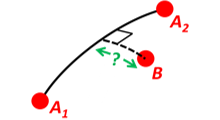Cross track distance (cross track error).

### Solution

Given

n_EA1_E <- lat_lon2n_E(rad(0), rad(0))

r_Earth <- 6371e3  # mean Earth radius (m)

Find the unit normal to the great circle between n_EA1_E and n_EA2_E

c_E <- unit(pracma::cross(n_EA1_E, n_EA2_E))

Find the great circle cross track distance

(s_xt <- (acos(pracma::dot(c_E, n_EB_E)) - pi / 2) * r_Earth)
#>  11117.8

Find the Euclidean cross track distance

(d_xt <- -pracma::dot(c_E, n_EB_E) * r_Earth)
#>  11117.79